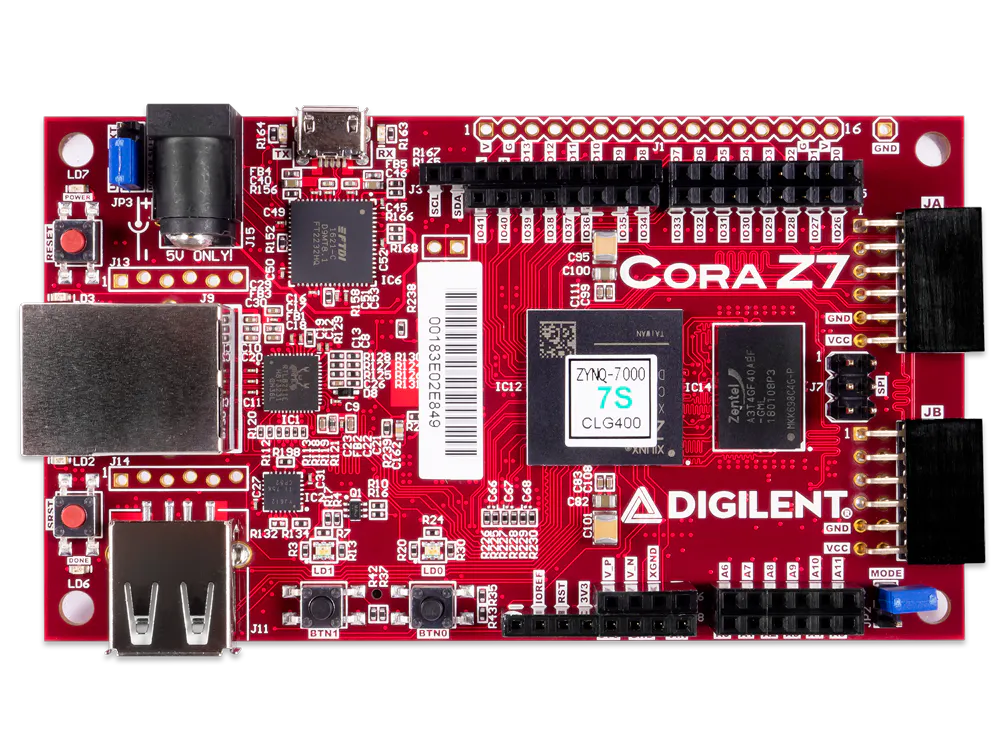# Estimating Pi with a Cora Z7 Running Linux

How should we go about figuring out how fast the Cora Z7 is? What about using both CPU cores? What about using a full OS?## Things used in this project

### Software apps and online servicesAMD PetaLinux

## Code

### main.go

Go
Go application source code. To be built for ARM architecture to run in a Cora Petalinux environment.
```package main

import (
"fmt"
"math/rand"
"runtime"
"time"
)

func PI(samples int) float64 {
var inside int = 0

r := rand.New(rand.NewSource(time.Now().UnixNano()))
for i := 0; i < samples; i++ {
x := r.Float64()
y := r.Float64()
if (x*x + y*y) < 1 {
inside++
}
}

ratio := float64(inside) / float64(samples)

return ratio * 4
}

func MultiPI(samples int) float64 {
cpus := runtime.NumCPU()

results := make(chan float64, cpus)

for j := 0; j < cpus; j++ {
go func() {
var inside int
r := rand.New(rand.NewSource(time.Now().UnixNano()))
for i := 0; i < threadSamples; i++ {
x, y := r.Float64(), r.Float64()

if x*x+y*y <= 1 {
inside++
}
}
results <- float64(inside) / float64(threadSamples) * 4
}()
}

var total float64
for i := 0; i < cpus; i++ {
total += <-results
}

}

func init() {
runtime.GOMAXPROCS(runtime.NumCPU())
}

func main() {
fmt.Println("Running Monte Carlo simulations ...\n")

var iterations = 524287500
fmt.Println(iterations, "iterations in a single thread")
start := time.Now()
fmt.Println("calculated", PI(iterations))
t := time.Now()
elapsed := t.Sub(start)
fmt.Println("in", elapsed)

fmt.Println()
fmt.Println(iterations, "iterations in a multiple threads")
start2 := time.Now()
fmt.Println("calculated", MultiPI(iterations))
t2 := time.Now()
elapsed2 := t2.Sub(start2)
fmt.Println("in", elapsed2)
}
```

## Credits

### Arthur Brown

14 projects • 30 followers
Applications engineer and digital logic geek

### Arvin Tang

2 projects • 5 followers

### Sam Kristoff

35 projects • 52 followers
R&D Director at NI

### Andrew Holzer

2 projects • 2 followers